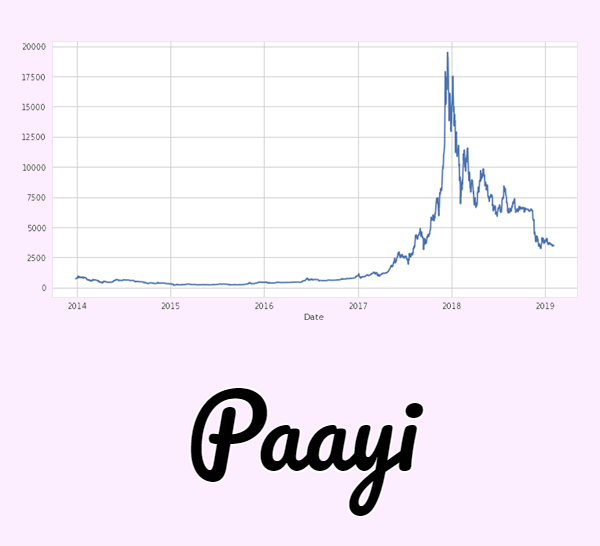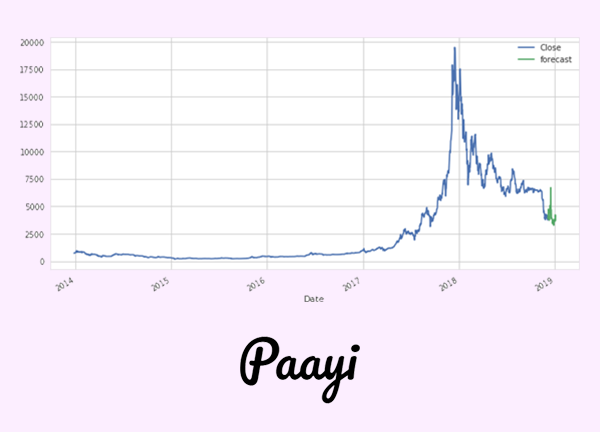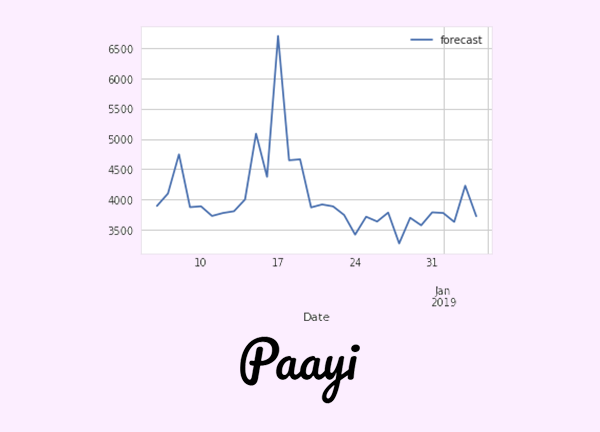## Boosting Technique in Python

##### In this part of Learning Python we Cover Boosting Technique In Python
Written by |08-May-2019 | 0 Comments | 185 Views

Drawbacks of Boosting:

• Boost will overfit the model by combining the weak learner.
• Very high training time.
• Training a face detector will take 2 weeks on a modern computer.

Discrete Ada boost is used as an improved variant of Ada boost. In which instead calculating the errors, we compute the probability of correct classification.

Bagging aggregates predictions of its base estimators and produces a final prediction. This is a measure designed to reduce over-fitting (variance) - however, this leads to an increase in bias, which is compensated for by the reduction in variance though.

Boosting combines several weak learners to give a single active learner by iteratively training the weak learners, then learning from their errors and misclassifications and improving on them further. This leads to an increase in variance but reduces bias significantly.

The variance-bias trade-off is up to the user.

Other Boosting Examples:

• Brown Boost: Brown boost is a boosting algorithm that is robust to the noisy data.
• So boosting: It can be used for semi-supervised learning in the case in which there is redundancy in features.
• LpBoost: Lp-Boost belongs to a boosting family. It maximizes the margin between training samples of the given data and hence also belongs to the class maximizing algorithm of supervised learning.

Real Life Applications of Ensemble Boosting:

• Image Recognition
• Credit Card Fraud
• Genes Classification
• Medical diagnoses

Conclusion:

Ensemble methods can help you win machine learning competitions by devising sophisticated algorithms and producing results with high accuracy, the effectiveness of these methods is undeniable, and their benefits in appropriate applications can be tremendous. In fields such as healthcare, even the smallest amount of improvement in the accuracy of machine learning algorithms can be something precious.

Project:

Now we will develop a small project in which we use two classifiers of decision tree family and will be using a regression technique to forecast the bitcoin price data.

This small project is available on my GitHub. The link is as follows:

https://github.com/HassanRehman11/BitcoinTimeSeries

So let's dig into the code.

`import pandas as pd`
`import imp`
`from sklearn import preprocessing`
`from sklearn.ensemble import RandomForestRegressor`
`from dateutil.parser import parse`
`import seaborn as sns`
`import matplotlib.pyplot as plt`
`from sklearn import cross_validation`
`from datetime import datetime, timedelta`

So first we import all the library that we will be using  in this code.

`df = pd.read_html('https://coinmarketcap.com/currencies/bitcoin/historical-data/?start=20130428&end=20190203')`

After that, we scrape the tabular data from the website using pandas read HTML. The data look like this.

 Date Open* High Low Close** Volume Market Cap 0 Feb 03, 2019 3516.14 3521.39 3447.92 3464.01 5043937584 60681847608 1 Feb 02, 2019 3484.63 3523.29 3467.57 3521.06 5071623601 61675119055 2 Feb 01, 2019 3460.55 3501.95 3431.59 3487.95 5422926707 61088747491 3 Jan 31, 2019 3485.41 3504.80 3447.92 3457.79 5831198271 60553903927 4 Jan 30, 2019 3443.90 3495.17 3429.39 3486.18 5955112627 61044262622 5 Jan 29, 2019 3468.87 3476.07 3400.82 3448.12 5897159493 60371874099 6 Jan 28, 2019 3584.28 3586.75 3439.23 3470.45 6908930483 60756570314 7 Jan 27, 2019 3604.69 3612.67 3567.25 3583.97 5570752966 62737274093 8 Jan 26, 2019 3599.72 3654.93 3593.35 3602.46 5098183235 63054898963 9 Jan 25, 2019 3607.39 3612.93 3575.60 3599.77 5265847539 63000985908 10 Jan 24, 2019 3584.50 3616.09 3569.09 3600.87 5262869046 63014066012 11 Jan 23, 2019 3605.56 3623.07 3565.31 3585.12 5433755649 62731361272 12 Jan 22, 2019 3575.08 3620.75 3539.72 3604.58 5313623556 63065139424 13 Jan 21, 2019 3600.37 3608.84 3558.54 3576.03 5004347059 62559869612 14 Jan 20, 2019 3725.45 3743.39 3583.02 3601.01 5582489560 62990143284 15 Jan 19, 2019 3652.38 3758.53 3652.38 3728.57 5955691380 65214103659 16 Jan 18, 2019 3677.99 3682.52 3637.08 3657.84 5002961727 63970991867 17 Jan 17, 2019 3651.87 3680.14 3621.96 3678.56 5464420383 64327048255 18 Jan 16, 2019 3631.51 3685.78 3624.67 3655.01 5394457145 63909348776 19 Jan 15, 2019 3704.22 3720.15 3619.95 3630.68 5537192302 63477817959 20 Jan 14, 2019 3557.31 3727.84 3552.29 3706.05 5651384490 64789619995 2

It contain the dates ,open price, highest price, closing price, and lowest price. Moreover, it contains volume and market cap.

After that, we will make the data clean because without cleaning the data our model will not be able to forecast correctly because there are many empty rows. We have to remove such rows and make the data more acceptable before feeding it to the algorithm.

`df = df[df['Volume']!='-']`
`df = df.reindex(index=df.index[::-1])`
`df['Volume'] = df['Volume'].astype(int)`
`df['Market Cap'] = df['Market Cap'].astype(int)`
`df = df.reset_index()`

If we look upon the data, we see few rows in the last have “-” value in the volume, so we have to remove these rows and change the type of volume and market cap to int to reduce somehow its dimensionality.

`df['Date'] = df['Date'].apply(lambda x:parse(str(x)).date())`
`df = df.set_index('Date')`
`df=df.drop('index', axis=1)`
`sns.set()`
`sns.set_style('whitegrid')`
`df['Close**'].plot(figsize=(12,6),label='Close')`
`plt.show()`

The date time is given as a string. The plotting will not recognize the string when we try to plot it on the x-axis. So before this, we first have to convert the string into proper date time format that python can understand.

After that, we set the date as an index. The plotting library will automatically understand that the index has to be on the x-axis. We then drop the old index and plot the closing of bitcoin. The graph looks like this.We can see that data starts in 2014 and ends in 2019 which is approximately 5 years of data.

`df['average'] = (df['Open*']+df['High']+df['Low']+df['Close**'])/4`
`df['shift'] = df['Close**'].shift(-60)`
`df.dropna(inplace=True)`
`X=df.drop('shift',axis=1)`
`y=df['shift']`
`X_train,X_test,y_train,y_test=cross_validation.train_test_split(X,y,test_size=0.2,random_state=7)`

Now we split the data. Now we will train the model on two decision tree — the first one in a random forest regression technique. Regression is because the data is continuous, not categorical.

`reg=RandomForestRegressor(n_estimators=400,random_state=7)`
`reg.fit(X_train,y_train)`
`accuracy=reg.score(X_test,y_test)`
`accuracy=accuracy*100`
`accuracy = float("{0:.4f}".format(accuracy))`
`print('Accuracy is:',accuracy,'%')`

`import xgboost as xgb`
`model = xgb.XGBRegressor(colsample_bytree=0.4603, gamma=0.0468, `
`                             learning_rate=0.01, max_depth=40, `
`                             min_child_weight=1.7817, n_estimators=400,`
`                             reg_alpha=0.4640, reg_lambda=0.8571,`
`                             subsample=0.5213, silent=1,`
`                             random_state =7, nthread = -1)`
`model.fit(X_train,y_train)`
`accuracy=model.score(X_test,y_test)`
`accuracy=accuracy*100`
`accuracy = float("{0:.4f}".format(accuracy))`
`print('Accuracy is:',accuracy,'%')`

The second one is the XG booster which is a boosting technique. The accuracy of random forest is 92, and XG boost is of 92%, which makes it a good classifier.

We now have to shift the data. The shifting of data will be of 30 days. So now we will shift the data.

`X_30=X[-30:]`
`forecast=model.predict(X_30)`

When the data is shifted than we predict this data.

`prev_date = df.iloc[-1].name`
`next_date = prev_date + timedelta(days=1)`
`date=pd.date_range(next_date,periods=30,freq='D')`
`df1=pd.DataFrame(forecast,columns=['Forecast'],index=date)`
`df1.index.name='Date'`

Now we will create the index of the next 30 days. Now we will predict the shifted data.

`df['Close**'].plot(figsize=(12,6),label='Close')`
`df1['Forecast'].plot(label='forecast')`
`plt.legend()``d0f1['Forecast'].plot(label='forecast')`
`plt.legend()`The green line is the forecasted.